|

# 林内携手国际顶级采暖辅材 助力中国采暖市场发展

7月25日，大暑节气刚过，采暖系统行业人士已经未雨绸缪，开始为人们冬天如何用上舒适、高品质的采暖进行精心准备。林内携手采暖系统领域的知名国际品牌---德国瑞好、丹麦丹佛斯、英国赫兰德，以及长三角舒适家居行业领先企业上海林适舒适家居公司，共同达成战略合作，建成采暖系统行业第一家整合了壁挂炉、系统辅材、设计安装维保等一站式舒适采暖服务平台，助力中国分户采暖市场发展。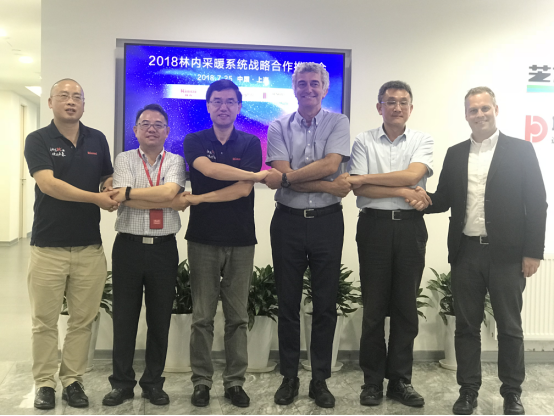随着人们生活和收入水平的提高，在中国淮河以南非传统意义上的集中供暖地区地区，壁挂炉分户供暖有着旺盛的市场需求。同时在北方地区，由于国家积极推进“蓝天工程”、“煤改气”等政策的持续、快速推进，我国分户采暖市场未来预计仍将呈现出高速增长。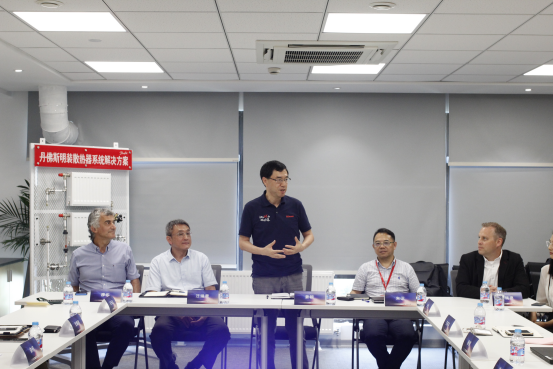业内资深人士透露，即便是我们许多耳熟能详的品牌，为了迎合当下爆发的分户采暖市场需求，对壁挂炉和系统设备的匹配性也还未完全掌握，缺乏专业产品技术和系统服务经验，而采暖系统的使用质保期长，单一的专业品牌，很难为消费者提供一系列专业、可靠、舒适、节能的一站式采暖系统解决方案服务。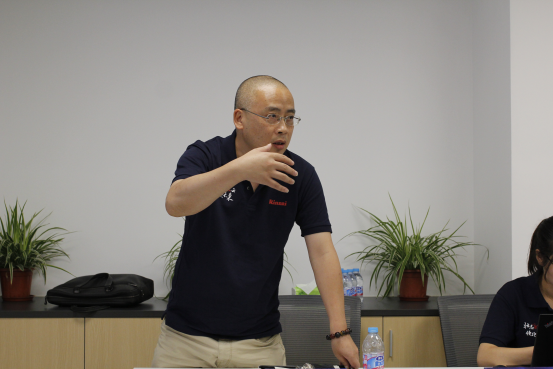据了解，就采暖整个系统而言，壁挂炉单品占采暖系统成本的1/3，其他2/3在于其他配套系统散热部份（地暖，或暖气片）、控制系统、施工和维保等成本，因此，壁挂炉单品在整个采暖系统成本的占比并不高，但整个壁挂炉加上采暖系统产品的配套、设计、施工和后续维保，对于最终用户使用的满意度有很重要影响。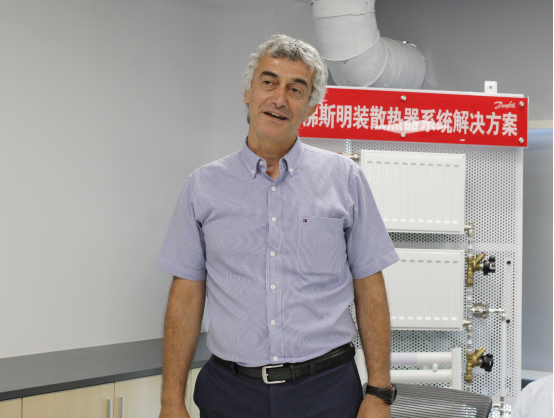为解决用户在采暖系统选购、安装、使用上的众多问题点，上海林内管理层经过多次专题研究和酝酿，今年开春就开启了与采暖系统领域多个国际知名品牌的战略合作洽谈。3月29日与德国瑞好公司就地暖系统配置达成战略合作；5月29日与英国赫兰德公司就暖气片选配达成战略合作；6月29日与丹麦丹佛斯公司签署采暖系统控制装置合作协议。未来，上海林内还将整合更多的采暖优势资源来服务中国的采暖用户，助力行业品质能力的全面提升。

众所周知，在我国壁挂炉市场快速增长的同时，也暴露出了一些问题让人隐忧。目前，市场上壁挂炉品牌、系统设备、系统辅材品牌众多，质量不一，且假冒伪劣产品鱼目混珠，产生了很多质量问题，给消费者选择造成了很多困扰，严重影响了消费者对于分户采暖的认知和使用。

采暖系统技术、施工相对比较专业，普通消费者对采暖设备、辅材等了解有限，特别是家庭采暖系统，需要消费者在安装时提前设计方案，合理布置好管路，一旦选择不当，会直接影响到日后的使用，可以说是后患无穷。为了让更多的消费者少走弯路，上海林内公司在原有与各大采暖系统包括管材、暖气片、温控器等企业合作提供一站式服务的基础上，推进建立战略合作伙伴关系，旨在直接对接这些企业的全球采购平台，获取更多的技术和资源支持，为用户提供更经济、可靠、安全、舒适、节能的系统产品，推动采暖行业的高水平发展。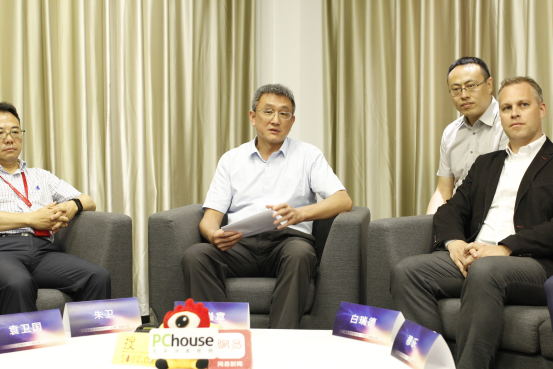战略会议结束后，搜狐家居有幸采访了埃瑟吉供热设备（上海）有限公司总经理穆乐。在双方合作带来的利好方面，穆总认为林内去选择各个行业顶尖的辅材供应商作为合作伙伴是源于对品质的坚持，这也是赫兰德一直对品质的坚持。

这次合作实际上给了双方一个非常好的平台，首先是赫兰德作为一个进入中国市场时间不久的公司，可以借助林内非常成熟的B2C平台进行更好地推广。赫兰德也有市面上最多款形的选项，对散热器来讲是非常大的优势，这个也可以作为推广的长处被林内所使用。

穆总现场还教与大家如何直接判断一个散热片的好坏。分两个方面，第一个方面是追本溯源。因为现在的终端用户，更多倾向上网寻求更多的信息了解品牌。赫兰德每一组散热器上都有对应的条形码，你可以通过扫描条形码获知它的工厂所在地，品牌所在地，以及我们每一组散热器都有一个像身份证号一样的编号，可以通过这一串编号在工厂系统查询到它的批次，生产日期。这不是每一个工厂可以做到的。

另一个方面，通过直观的看、摸，都可以简单判断一组散热器的品质好坏，包括它的焊点，包括它的表面的一些喷漆的处理，以及它的终端是不是光滑等等，这些都是可以作为判断散热器质量好坏的一些要点。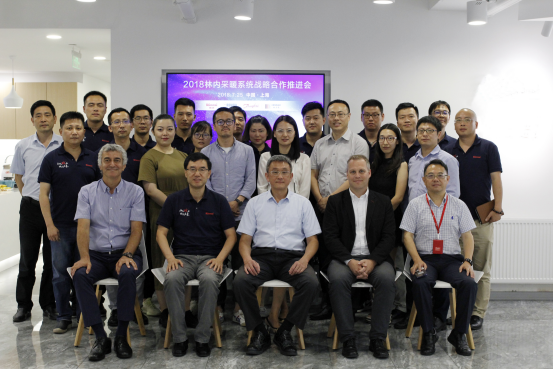林内进入中国市场25年来，如今已非常了解中国各区域的采暖特点和用户的切身需求，通过与德国瑞好、丹麦丹佛斯、英国赫兰德的深度合作，将全球最优质的系统资源合作提升到战略合作层面，无疑是在深耕中国市场，给消费者带来可靠的、高品质采暖系统解决方案和一站式的设计、安装、售后服务。同时，这一战略合作还将提升整个行业对于产品服务和品质的关注，推动采暖行业向专业化和一体化的发展，为采暖行业一体化服务树立了新标杆。

`声明：本文由入驻焦点开放平台的作者撰写，除焦点官方账号外，观点仅代表作者本人，不代表焦点立场错误信息举报电话： 400-099-0099，邮箱：jubao@vip.sohu.com，或点此进行意见反馈，或点此进行举报投诉。`A B C D E F G H J K L M N P Q R S T W X Y Z
A - B - C - D - E
• A
• 鞍山
• 安庆
• 安阳
• 安顺
• 安康
• 澳门
• B
• 北京
• 保定
• 包头
• 巴彦淖尔
• 本溪
• 蚌埠
• 亳州
• 滨州
• 北海
• 百色
• 巴中
• 毕节
• 保山
• 宝鸡
• 白银
• 巴州
• C
• 承德
• 沧州
• 长治
• 赤峰
• 朝阳
• 长春
• 常州
• 滁州
• 池州
• 长沙
• 常德
• 郴州
• 潮州
• 崇左
• 重庆
• 成都
• 楚雄
• 昌都
• 慈溪
• 常熟
• D
• 大同
• 大连
• 丹东
• 大庆
• 东营
• 德州
• 东莞
• 德阳
• 达州
• 大理
• 德宏
• 定西
• 儋州
• 东平
• E
• 鄂尔多斯
• 鄂州
• 恩施
F - G - H - I - J
• F
• 抚顺
• 阜新
• 阜阳
• 福州
• 抚州
• 佛山
• 防城港
• G
• 赣州
• 广州
• 桂林
• 贵港
• 广元
• 广安
• 贵阳
• 固原
• H
• 邯郸
• 衡水
• 呼和浩特
• 呼伦贝尔
• 葫芦岛
• 哈尔滨
• 黑河
• 淮安
• 杭州
• 湖州
• 合肥
• 淮南
• 淮北
• 黄山
• 菏泽
• 鹤壁
• 黄石
• 黄冈
• 衡阳
• 怀化
• 惠州
• 河源
• 贺州
• 河池
• 海口
• 红河
• 汉中
• 海东
• I
• J
• 晋中
• 锦州
• 吉林
• 鸡西
• 佳木斯
• 嘉兴
• 金华
• 景德镇
• 九江
• 吉安
• 济南
• 济宁
• 焦作
• 荆门
• 荆州
• 江门
• 揭阳
• 金昌
• 酒泉
• 嘉峪关
K - L - M - N - P
• K
• 开封
• 昆明
• 昆山
• L
• 廊坊
• 临汾
• 辽阳
• 连云港
• 丽水
• 六安
• 龙岩
• 莱芜
• 临沂
• 聊城
• 洛阳
• 漯河
• 娄底
• 柳州
• 来宾
• 泸州
• 乐山
• 六盘水
• 丽江
• 临沧
• 拉萨
• 林芝
• 兰州
• 陇南
• M
• 牡丹江
• 马鞍山
• 茂名
• 梅州
• 绵阳
• 眉山
• N
• 南京
• 南通
• 宁波
• 南平
• 宁德
• 南昌
• 南阳
• 南宁
• 内江
• 南充
• P
• 盘锦
• 莆田
• 平顶山
• 濮阳
• 攀枝花
• 普洱
• 平凉
Q - R - S - T - W
• Q
• 秦皇岛
• 齐齐哈尔
• 衢州
• 泉州
• 青岛
• 清远
• 钦州
• 黔南
• 曲靖
• 庆阳
• R
• 日照
• 日喀则
• S
• 石家庄
• 沈阳
• 双鸭山
• 绥化
• 上海
• 苏州
• 宿迁
• 绍兴
• 宿州
• 三明
• 上饶
• 三门峡
• 商丘
• 十堰
• 随州
• 邵阳
• 韶关
• 深圳
• 汕头
• 汕尾
• 三亚
• 三沙
• 遂宁
• 山南
• 商洛
• 石嘴山
• T
• 天津
• 唐山
• 太原
• 通辽
• 铁岭
• 泰州
• 台州
• 铜陵
• 泰安
• 铜仁
• 铜川
• 天水
• 天门
• W
• 乌海
• 乌兰察布
• 无锡
• 温州
• 芜湖
• 潍坊
• 威海
• 武汉
• 梧州
• 渭南
• 武威
• 吴忠
• 乌鲁木齐
X - Y - Z
• X
• 邢台
• 徐州
• 宣城
• 厦门
• 新乡
• 许昌
• 信阳
• 襄阳
• 孝感
• 咸宁
• 湘潭
• 湘西
• 西双版纳
• 西安
• 咸阳
• 西宁
• 仙桃
• 西昌
• Y
• 运城
• 营口
• 盐城
• 扬州
• 鹰潭
• 宜春
• 烟台
• 宜昌
• 岳阳
• 益阳
• 永州
• 阳江
• 云浮
• 玉林
• 宜宾
• 雅安
• 玉溪
• 延安
• 榆林
• 银川
• Z
• 张家口
• 镇江
• 舟山
• 漳州
• 淄博
• 枣庄
• 郑州
• 周口
• 驻马店
• 株洲
• 张家界
• 珠海
• 湛江
• 肇庆
• 中山
• 自贡
• 资阳
• 遵义
• 昭通
• 张掖
• 中卫

1室1厅1厨1卫1阳台

1
2
3
4
5

0
1
2

1

1

0
1
2
3报名成功，资料已提交审核A B C D E F G H J K L M N P Q R S T W X Y Z
A - B - C - D - E
• A
• 鞍山
• 安庆
• 安阳
• 安顺
• 安康
• 澳门
• B
• 北京
• 保定
• 包头
• 巴彦淖尔
• 本溪
• 蚌埠
• 亳州
• 滨州
• 北海
• 百色
• 巴中
• 毕节
• 保山
• 宝鸡
• 白银
• 巴州
• C
• 承德
• 沧州
• 长治
• 赤峰
• 朝阳
• 长春
• 常州
• 滁州
• 池州
• 长沙
• 常德
• 郴州
• 潮州
• 崇左
• 重庆
• 成都
• 楚雄
• 昌都
• 慈溪
• 常熟
• D
• 大同
• 大连
• 丹东
• 大庆
• 东营
• 德州
• 东莞
• 德阳
• 达州
• 大理
• 德宏
• 定西
• 儋州
• 东平
• E
• 鄂尔多斯
• 鄂州
• 恩施
F - G - H - I - J
• F
• 抚顺
• 阜新
• 阜阳
• 福州
• 抚州
• 佛山
• 防城港
• G
• 赣州
• 广州
• 桂林
• 贵港
• 广元
• 广安
• 贵阳
• 固原
• H
• 邯郸
• 衡水
• 呼和浩特
• 呼伦贝尔
• 葫芦岛
• 哈尔滨
• 黑河
• 淮安
• 杭州
• 湖州
• 合肥
• 淮南
• 淮北
• 黄山
• 菏泽
• 鹤壁
• 黄石
• 黄冈
• 衡阳
• 怀化
• 惠州
• 河源
• 贺州
• 河池
• 海口
• 红河
• 汉中
• 海东
• I
• J
• 晋中
• 锦州
• 吉林
• 鸡西
• 佳木斯
• 嘉兴
• 金华
• 景德镇
• 九江
• 吉安
• 济南
• 济宁
• 焦作
• 荆门
• 荆州
• 江门
• 揭阳
• 金昌
• 酒泉
• 嘉峪关
K - L - M - N - P
• K
• 开封
• 昆明
• 昆山
• L
• 廊坊
• 临汾
• 辽阳
• 连云港
• 丽水
• 六安
• 龙岩
• 莱芜
• 临沂
• 聊城
• 洛阳
• 漯河
• 娄底
• 柳州
• 来宾
• 泸州
• 乐山
• 六盘水
• 丽江
• 临沧
• 拉萨
• 林芝
• 兰州
• 陇南
• M
• 牡丹江
• 马鞍山
• 茂名
• 梅州
• 绵阳
• 眉山
• N
• 南京
• 南通
• 宁波
• 南平
• 宁德
• 南昌
• 南阳
• 南宁
• 内江
• 南充
• P
• 盘锦
• 莆田
• 平顶山
• 濮阳
• 攀枝花
• 普洱
• 平凉
Q - R - S - T - W
• Q
• 秦皇岛
• 齐齐哈尔
• 衢州
• 泉州
• 青岛
• 清远
• 钦州
• 黔南
• 曲靖
• 庆阳
• R
• 日照
• 日喀则
• S
• 石家庄
• 沈阳
• 双鸭山
• 绥化
• 上海
• 苏州
• 宿迁
• 绍兴
• 宿州
• 三明
• 上饶
• 三门峡
• 商丘
• 十堰
• 随州
• 邵阳
• 韶关
• 深圳
• 汕头
• 汕尾
• 三亚
• 三沙
• 遂宁
• 山南
• 商洛
• 石嘴山
• T
• 天津
• 唐山
• 太原
• 通辽
• 铁岭
• 泰州
• 台州
• 铜陵
• 泰安
• 铜仁
• 铜川
• 天水
• 天门
• W
• 乌海
• 乌兰察布
• 无锡
• 温州
• 芜湖
• 潍坊
• 威海
• 武汉
• 梧州
• 渭南
• 武威
• 吴忠
• 乌鲁木齐
X - Y - Z
• X
• 邢台
• 徐州
• 宣城
• 厦门
• 新乡
• 许昌
• 信阳
• 襄阳
• 孝感
• 咸宁
• 湘潭
• 湘西
• 西双版纳
• 西安
• 咸阳
• 西宁
• 仙桃
• 西昌
• Y
• 运城
• 营口
• 盐城
• 扬州
• 鹰潭
• 宜春
• 烟台
• 宜昌
• 岳阳
• 益阳
• 永州
• 阳江
• 云浮
• 玉林
• 宜宾
• 雅安
• 玉溪
• 延安
• 榆林
• 银川
• Z
• 张家口
• 镇江
• 舟山
• 漳州
• 淄博
• 枣庄
• 郑州
• 周口
• 驻马店
• 株洲
• 张家界
• 珠海
• 湛江
• 肇庆
• 中山
• 自贡
• 资阳
• 遵义
• 昭通
• 张掖
• 中卫• 手机• 分享
• 设计
免费设计
• 计算器
装修计算器
• 入驻
合作入驻
• 联系
联系我们
• 置顶
返回顶部